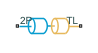# Interface (2P-TL)

Two-phase fluid and thermal liquid network interface

Since R2021a

•Libraries:
Simscape / Fluids / Fluid Network Interfaces

## Description

The Interface (2P-TL) block represents a flow connection between a two-phase fluid network and a thermal liquid network. Pressure, temperature, and mass flow rate are equal at the interface. The block does not transfer the fluid properties of each fluid network across the interface. To conserve fluid properties, ensure that the Thermal Liquid Properties (TL) block and the Two-Phase Fluid Properties (2P) block have the same settings.

The fluid at the 2P port must remain subcooled. You can use the Two-phase fluid subcooled liquid check parameter to choose what action the block performs if the fluid does not meet this condition.

### Mass and Momentum Balance

The block conserves mass such that

`${\stackrel{˙}{m}}_{TL}+{\stackrel{˙}{m}}_{2P}=0,$`

where:

• ${\stackrel{˙}{m}}_{TL}$ is the mass flow rate into port TL.

• ${\stackrel{˙}{m}}_{2P}$ is the mass flow rate into port 2P.

The port pressures are equal:

`${P}_{TL}={P}_{2P}.$`

### Energy Balance

The temperature is constant between the two fluid ports. The block calculates the energy balance over the interface as

`${\Phi }_{TL}+{\Phi }_{2P}={\stackrel{˙}{m}}_{TL}\left({u}_{TL}+{p}_{TL}{v}_{TL}+\frac{{w}_{TL}^{2}}{2}\right)+{\stackrel{˙}{m}}_{2P}\left({u}_{2P}+{p}_{2P}{v}_{2P}+\frac{{w}_{2P}^{2}}{2}\right),$`

where:

• ΦTL is the energy flow rate into port TL.

• Φ2P is the energy flow rate into port 2P.

• hTL is the specific internal energy at port TL.

• h2P is the specific internal energy at port 2P.

• vTL is the specific volume at port TL.

• v2P is the specific volume at port 2P.

• wTL is the flow velocity at port TL

`${w}_{TL}=\frac{{\stackrel{˙}{m}}_{TL}{v}_{TL}}{S},$`

• w2P is the flow velocity at port 2P

`${w}_{2P}=\frac{{\stackrel{˙}{m}}_{2P}{v}_{2P}}{S}.$`

• S is the value of the Cross-sectional area at ports 2P and TL parameter.

## Ports

### Conserving

expand all

Two-phase fluid conserving port associated with the two-phase fluid at the interface.

Thermal liquid conserving port associated with the thermal liquid at the interface.

## Parameters

expand all

Cross-sectional area of the thermal liquid and two-phase fluid ports.

Action to perform when the fluid at port 2P does not meet subcooled conditions.

## Version History

Introduced in R2021a